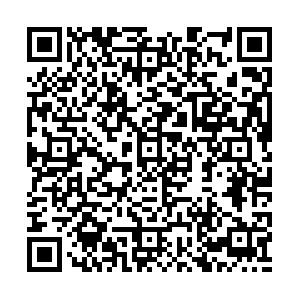## 留言板引用本文: 王雪松, 刘卫东, 刘典. 新型反四手性蜂窝结构的面内拉伸弹性[J]. 复合材料学报, 2023, 40(8): 4854-4866WANG Xuesong, LIU Weidong, LIU Dian. In-plane tensile elasticity of a novel anti-tetrachiral cellular structure[J]. Acta Materiae Compositae Sinica, 2023, 40(8): 4854-4866.
 Citation: WANG Xuesong, LIU Weidong, LIU Dian. In-plane tensile elasticity of a novel anti-tetrachiral cellular structure[J]. Acta Materiae Compositae Sinica, 2023, 40(8): 4854-4866.## 新型反四手性蜂窝结构的面内拉伸弹性

###### 通讯作者: 刘卫东，博士，副教授，研究方向为机械结构设计与理论　E-mail：liuweidong@hhu.edu.cn
• 中图分类号: O342;V257

## In-plane tensile elasticity of a novel anti-tetrachiral cellular structure

Funds: National Natural Science Foundation of China(51605140)
• 摘要: 手性蜂窝结构不仅具有蜂窝结构轻质高强、高柔韧性等优点，同时还拥有负泊松比蜂窝的拉胀特性，在新型柔性结构方面受到国内外众多学者的广泛研究。但传统手性蜂窝结构通常具有刚性中心节点，且以切向直梁进行连接，虽具有较高的刚度，但刚性节点的存在也削弱了结构的变形能力，限制了其在具有高柔性需求场合的应用。本文提出一种由半周期正弦梁组成的新型反四手性蜂窝结构，该结构舍弃了中心刚性节点，单纯以梁结构进行连接，同时将直梁替换为特殊曲梁，缓解了结构在节点处的应力集中问题，结构简单且性能可调。因此，新型反四手性蜂窝具有更加优越的变形能力，等效弹性模量比传统手性结构低2~3个数量级，等效负泊松比的变化范围也扩大了近2倍，在船舶、医疗、航空航天等领域前景广阔。正弦半周期型反四手性结构与传统手性结构随相对密度${\rho _{\text{r}}}$变化的${E_{\text{x}}}/E$(a)和${\nu _{{\text{xy}}}}$(b)性能比较(传统手性结构：$r = 5{\text{mm}}$${L_{\text{x}}} = {L_{\text{y}}} = 20{\text{mm}}$；正弦半周期型结构：$H = L = 20{\text{mm}}$)

• 图  1  半周期正弦梁反四手性蜂窝结构

Figure  1.  Anti-tetrachiral cellular structure of half-periodic sine beams

图  2  半周期正弦梁蜂窝单元结构参数

Figure  2.  Structural parameters of unit cell with half-periodic sine beams

图  3  反四手性蜂窝结构沿x方向单轴拉伸

Figure  3.  Uniaxial stretching along the x-direction of the anti-tetrachiral cellular structure

${\sigma _x}$ is the equivalent stress along the x-direction.${P_x}$ and ${P_y}$ are the tensile loads along the x- and y-directions, respectively; ${S_x}$ and ${S_y}$ are the shear loads along the x- and the y-directions，respectively; ${M_1}$ and ${M_2}$ are the interacting bending moments of the cross sections

图  4  反四手性蜂窝结构有限元仿真模型

Figure  4.  Finite element model of the anti-tetrachiral cellular structure

图  5  反四手性蜂窝结构等效弹性模量${E_x}/E$随参数$\xi$$\varphi$变化的理论预测和仿真结果

Figure  5.  Theoretical predictions and FE results for the equivalent elastic modulus ${E_x}/E$of the anti-tetrachiral cellular structure varying with $\xi$and $\varphi$图  6  反四手性蜂窝结构等效泊松比${\nu _{{\text{xy}}}}$随参数$\xi$$\varphi$变化的仿真结果和理论值预测

Figure  6.  Theoretical predictions and FE results for the equivalent Poisson’s ratio ${\nu _{{\text{xy}}}}$of the anti-tetrachiral cellular structure varying with $\xi$and $\varphi$图  7  单轴载荷下变形前后反四手性蜂窝结构等效应力图

Figure  7.  Equivalent stress diagram of the anti-tetrachiral cellular structure before and after deformation under uniaxial loads

图  8  单轴载荷下变形后反四手性蜂窝结构z向转角图

Figure  8.  z-direction rotation angle diagram of the anti-tetrachiral cellular structure after deformation under uniaxial loads

图  9  3 D打印反四手性蜂窝结构试件

Figure  9.  3 D printed anti-tetrachiral cellular structure specimens

图  10  实验流程示意图

Figure  10.  Schematic diagram of the experimental test

图  11  反四手性蜂窝结构连接工艺示意图

Figure  11.  Schematic diagram of the joining process of anti-tetrachiral cellular structure

图  12  传统手性蜂窝结构

Figure  12.  Conventional chiral structure

图  13  正弦半周期型反四手性蜂窝结构与传统手性结构在不同壁厚下随相对密度${\rho _{\text{r}}}$变化的性能比较(传统手性结构：$r = 5{\text{mm}}$${L_x} = {L_y} = 20{\text{mm}}$；正弦半周期型结构：$H = L = 20{\text{mm}}$)

Figure  13.  Comparison of the properties between the half-periodic sine anti-tetrachiral cellular structure and conventional chiral structures under different thickness varying with relative density ${\rho _{\text{r}}}$(Conventional chiral structure:$r = 5{\text{mm}}$,${L_x} = {L_y} = 20{\text{mm}}$;half-periodic sine anti-tetrachiral structure:$H = L = 20{\text{mm}}$)

表  1  几何参数及无量纲参数设定

Table  1.   Geometric parameters and dimensionless parameters setting

 Geometric parameter Dimensionless parameter $L$ Spanwise length of the half-period sine beam along the horizontal direction $\alpha = L/H$ Aspect ratio ${V_{\text{L}}}$ Chordwise height of the half-period sine beam along the horizontal direction $\beta = L/H$ Thickness ratio $H$ Spanwise length of the half-period sine beam along the vertical direction $\gamma = b/H$ Gauge thickness ratio ${V_{\text{H}}}$ Chordwise height of the half-period sine beam along the vertical direction $\xi = {V_{\text{L}}}/H$ Chord ratio of the horizontal beam $t$ Thickness of the beams $\varphi = {V_{\text{H}}}/H$ Chord ratio of the vertical beam $b$ Gauge thickness

表  2  有限元仿真周期条件和边界条件

Table  2.   Periodic conditions and boundary conditions for finite element simulation

 Condition Uniaxial loading along the x-direction Periodiccondition ${u_x}\left( {\text{E}} \right) = {u_x}\left( {\text{F}} \right)$ ${u_x}\left( {\text{E}} \right) = {u_x}\left( {\text{F}} \right)$${\theta _z}\left( {\text{A}} \right) = {\theta _z}\left( {\text{B}} \right) {\theta _z}\left( {\text{C}} \right) = {\theta _z}\left( {\text{D}} \right)$${\theta _z}\left( {\text{E}} \right) = {\theta _z}\left( {\text{F}} \right)$ ${\theta _z}\left( {\text{G}} \right) = {\theta _z}\left( {\text{H}} \right)$ Boundary condition ${u_x}\left( {\text{A}} \right) = 0$${u_x}\left( {\text{C}} \right) = 0 {u_y}\left( {\text{C}} \right) = 0$${u_x}\left( {\text{B}} \right) = {\varepsilon _x} \times 4 L$${u_x}\left( {\text{D}} \right) = {\varepsilon _x} \times 4 L$ Notes: ${u_x}$ is the displacement along the x-direction; ${u_y}$ is the displacement along the y-direction; ${\theta _z}$ is the angle around of the z-axis; ${\varepsilon _x}$ is the strain along the x-direction.

表  3  实验与理论值的比较

Table  3.   Comparison between the experimental and theoretical results

 Number Parameters of the unit($H = L = 20{\text{mm}}$) ${E_x}/E$(Theoretical value) ${E_x}/E$(Experiment value) Error ① $\begin{gathered} \xi = \varphi = 0.5 \\ t = 1{\text{mm}} \\ \end{gathered}$ $4.13 \times {10^{ - 5}}$ $3.74 \times {10^{ - 5}}$ $9.4\%$ ② $\begin{gathered} \xi = \varphi = 0.5 \\ t = 2{\text{mm}} \\ \end{gathered}$ $3.29 \times {10^{ - 4}}$ $2.99 \times {10^{ - 4}}$ $9.1\%$ ③ $\begin{gathered} \xi = \varphi = 0.5 \\ t = 3{\text{mm}} \\ \end{gathered}$ $1.10 \times {10^{ - 3}}$ $9.98 \times {10^{ - 4}}$ $9.3\%$ ④ $\begin{gathered} \xi = 0.3,\varphi = 0.5 \\ t = 2{\text{mm}} \\ \end{gathered}$ $1.01 \times {10^{ - 4}}$ $9.21 \times {10^{ - 4}}$ $8.8\%$ ⑤ $\begin{gathered} \xi = 0.8,\varphi = 0.5 \\ t = 2{\text{mm}} \\ \end{gathered}$ $1.08 \times {10^{ - 4}}$ $1.00 \times {10^{ - 4}}$ $7.4\%$ ⑥ $\begin{gathered} \xi = 0.5,\varphi = 0.3 \\ t = 2{\text{mm}} \\ \end{gathered}$ $3.37 \times {10^{ - 4}}$ $3.07 \times {10^{ - 4}}$ $8.9\%$ ⑦ $\begin{gathered} \xi = 0.5,\varphi = 0.8 \\ t = 2{\text{mm}} \\ \end{gathered}$ $3.18 \times {10^{ - 4}}$ $2.92 \times {10^{ - 4}}$ $8.2\%$

表  4  传统手性蜂窝几何参数

Table  4.   Geometric parameters of conventional chiral structures

 Geometric parameter Dimensionless parameter $r$ Radius of central rigid node ${\alpha _x} = {L_x}/r$ Transverse beam ratio ${L_x}$ Length of transverse tangential ligaments ${\alpha _y} = {L_y}/r$ Longitudinal beam ratio ${L_y}$ Length of longitudinal tangential ligaments $\beta = t/r$ Thickness ratio $t$ Thickness of the beams $\gamma = b/r$ Gauge thickness ratio $b$ Gauge thickness
•点击查看大图
##### 计量
• 文章访问数:  264
• HTML全文浏览量:  214
• PDF下载量:  7
• 被引次数: 0
##### 出版历程
• 收稿日期:  2022-09-01
• 修回日期:  2022-10-17
• 录用日期:  2022-10-28
• 网络出版日期:  2022-11-11
• 刊出日期:  2023-08-15

### 目录/下载:  全尺寸图片 幻灯片
• 分享
• 用微信扫码二维码

分享至好友和朋友圈